# Test: Progression (AP And GP)- 2

## 10 Questions MCQ Test Quantitative Aptitude for Banking Preparation | Test: Progression (AP And GP)- 2

Description
Attempt Test: Progression (AP And GP)- 2 | 10 questions in 10 minutes | Mock test for UPSC preparation | Free important questions MCQ to study Quantitative Aptitude for Banking Preparation for UPSC Exam | Download free PDF with solutions
QUESTION: 1

### Find  the  15th  term  of  an  arithmetic  progression  whose  first  term  is  2  and  the  common  difference is 3

Solution: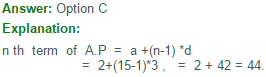QUESTION: 2

### What  is  the  sum  of  the  first  15  terms  of  an  A.P  whose  11 th  and   7 th  terms  are  5.25  and  3.25  respectively

Solution: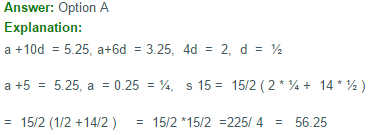QUESTION: 3

### If(12+22+32+…..+102)=385,then the value of (22+42+62 + …+202) is :

Solution:

(12+22+32+.......102)=385

(22+42+62+.......+202) = 22(12+22+ 32+.....+102 )
=4(385)
=1540

QUESTION: 4

In an arithmetic series consisting of 51 terms, the sum of the first three terms is 65 and the sum of the middle three terms is 129. What is the first term and the common difference of the series?

Solution: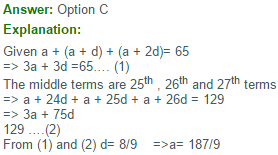QUESTION: 5

The sum of the first 100 numbers, 1 to 100 is divisible by

Solution: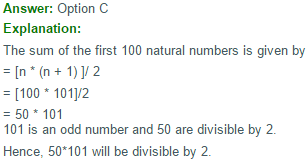QUESTION: 6

How many terms are there in G.P 3,6,12,24,….,384?

Solution: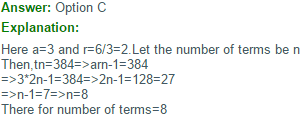QUESTION: 7

Four  angles  of  a  quadrilateral  are  in  G.P.  Whose  common  ratio  is  an  intiger.  Two  of  the  angles  are  acute  while  the  other  two  are  obtuse.   The  measure  of  the  smallest   angle  of  the  quadrilateral  is

Solution: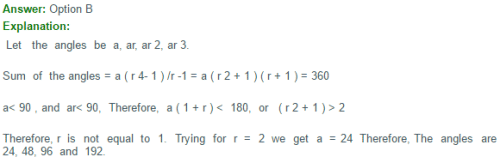QUESTION: 8

How many numbers between 11 and 90 divisible by 7?

Solution: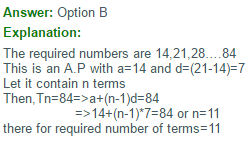QUESTION: 9

If Sn denotes the sum of the first n terms in an Arithmetic Progression and S1: S4 = 1: 10

Then the ratio of first term to fourth term is:

Solution: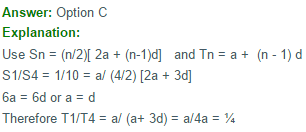QUESTION: 10

The sum of the three numbers in A.P is 21 and the product of their extremes is 45. Find the numbers.

Solution:

Let the numbers are be a - d, a, a + d
Then a - d + a + a + d = 21
3a = 21
a = 7
and (a - d)(a + d) = 45
a2 - d2 = 45
d2 = 4
d = +2
Hence, the numbers are 5, 7 and 9 when d = 2 and 9, 7 and 5 when d = -2. In both the cases numbers are the same.Use Code STAYHOME200 and get INR 200 additional OFF Use Coupon Code